# Difference between revisions of "Binomial heap"

The binomial heap or binomial queue is a heap data structure that implements all priority queue operations in worst-case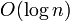$O(\log n)$ time (under the assumption of constant time to compare keys) and is desirable for its support for efficient merging (two binomial heaps of sizes$m$ and$n$ can be merged in$O(\log(m+n))$ time).

We follow Sedgewick's presentation of this data structure, which is isomorphic to but easier to understand than the version presented in the original 1978 paper by Vuillemin. (See section Binomial tree representation below.)

## Structure of power-of-two tree

A binomial heap is composed of zero or more power-of-two trees of unequal sizes. Each node of each power-of-two tree is labelled with one of the elements in the heap. A power-of-two tree has the following properties:

• It is a binary tree.
• Every node's label is greater than or equal to the labels of all nodes in its left subtree, but not necessarily its right. (This is a relaxation of the max-heap property.)
• The root has no right child.
• The root's left subtree is a complete binary tree with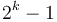$2^k-1$ nodes, where$k$ is a non-negative integer.

Therefore, a power-of-two tree of height$k$ has a total of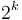$2^k$ nodes, and since the root has all other nodes in its left subtree, it must be the greatest node in the tree.

## Merging and splitting power-of-two trees

A power-of-two tree with$2^k$ nodes, where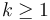$k \geq 1$, can be split into two power-of-two trees of size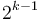$2^{k-1}$ each, as follows. If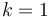$k = 1$, then it is fairly obvious how to do this. Otherwise, call the root of the tree R; call R's left child P; and call P's right child Q. Now make Q the left child of R, so that P is no longer the left child of R, and P no longer has a right child. Now that P no longer has a right child, it is a power-of-two tree of size$2^{k-1}$. Also, because the subtree rooted at Q was initially part of R's left subtree, when Q is made the left child of R, the left-heap-ordering property continues to hold, so R's tree is still a valid power-of-two tree, again of size$2^{k-1}$. This procedure takes constant time.

Merging two power-of-two trees with size$2^k$ to give one power-of-two tree with size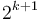$2^{k+1}$ is the reverse process. If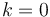$k=0$, we simply make the node with the smaller label the left child of the node with the larger label. Otherwise, call the two roots P and Q, and their left children R and S, respectively. Then, if P's label is greater than or equal to Q's, then P is destined to become the root of the merged tree; we make Q its left child, and make R the right child of Q. R's label might be greater than Q's, but that's okay, since R is the right child of Q, not the left. (This is why this relaxation is so important; it allows this step to be performed in constant time, plus the cost of one comparison, which is usually also constant.) If Q's label is greater, we instead make P the left child of Q, and S the right child of P.

## Structure of binomial heap

Since a binomial heap is only allowed to contain at most one power-of-two tree of each size, the structure of a binomial heap is uniquely determined by the binary representation of its size. For example, if a binomial heap contains 13 elements, that is, 11012, then we see that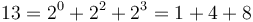$13 = 2^0 + 2^2 + 2^3 = 1 + 4 + 8$, so a binomial heap of size 13 contains three power-of-two trees, of sizes 1, 4, and 8, respectively.

It follows that the number of power-of-two trees in a binomial heap is no more than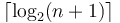$\lceil\log_2(n+1)\rceil$, since that is the length of the number$n$ expressed as a bit string.

## Finding the maximum

Since the root of each power-of-two tree is its greatest element, the greatest element in the entire heap must be the root of one of the trees. So all we have to do is check each root; the greatest root is then the greatest element in the entire heap. This requires only$O(\log n)$ comparisons.

## Merging

Let two binomial heaps be denoted$A$ and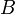$B$, with sizes$n$ and$m$, respectively. Let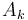$A_k$ denote$A$'s power-of-two tree of size$2^k$, if it has one, and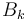$B_k$ denote likewise a power-of-two tree of$B$. We want to create a new binomial heap$S$ that contains all the nodes from either$A$ or$B$ and has size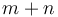$m+n$. After this operation,$A$ and$B$ will no longer exist as binomial heaps.

(In theory, we could create a new binomial heap$S$ that contained all the elements from both$A$ and$B$ without also destroying$A$ and$B$. However, this would require copying over all the data from both heaps, which would take linear time (in the sum of their sizes). This would then be no more efficient than simply using the binary heaps. For this reason, we assume we usually do not want to do this.)

The merge procedure is analogous to adding together two binary numbers.

We can think of the addition of binary numbers as being the shuffling around of bits, with the caveat that if we have too many bits, we have to perform a carry. We start from the rightmost column (the one's place), then proceed to the left, passing through the two's place, the four's place, and so on. At each step, we remember the carry bit from the previous step; this is initially zero, since there is no column to the right of the rightmost column to have produced a carry. Then, if we call the bits from the two numbers A and B, and the carry bit C, we do the following:

1. If A, B, and C are all zeroes, then the sum will contain a zero in this column, too; we don't have any bits to distribute.
2. If exactly one of A, B, and C is a one, and the other two are zeroes, then this bit is transferred into the sum; that is, the sum has a one in this column. The carry bit will be zero after this step, since there are no bits left over from this column.
3. If exactly two of A, B, and C are ones, then we don't have room in this column for both bits, so we have to exchange them for a single bit in the column to the left, and leave this column empty. That is, the carry bit is set to one, and the sum contains a zero in this column. This works because a set bit in column$k$ (where 0 represents the rightmost column) represents the value$2^k$. Adding two of these gives$2^{k+1}$, which is equivalent to a set bit in the next column to the left.
4. if A, B, and C are all ones, then there are three bits that want to be in this column, but only room for two; so two of them are exchanged for a single bit in the column to the left (the carry bit), and the third gets to occupy the current column (so the sum contains a one in this column).

Eventually, we exhaust all bits in both original numbers, and if there is still a carry bit, it is copied into the leftmost column of the sum, and the procedure terminates.

When merging two binomial heaps, we merge them starting from the "rightmost column", that is, the power-of-two trees of size one, and then proceed to the power-of-two trees of size two, and then size four, and so on. A nonexistent power-of-two tree of size$2^k$ corresponds to a zero in the$k$th column from the right, and an existent tree corresponds to a one. Carrying—that is, exchanging two ones in a given column for a one in the next column on the right—corresponds to merging two power-of-two trees of size$2^k$ to give a single power-of-two tree of size$2^{k+1}$. Intuitively, we merge whenever we have multiple power-of-two trees of a given size on our hands; there can only be one power-of-two tree of each size in the final sum. This is just like how we have to carry when adding binary numbers because there can only be one one in each column, so to speak.

This gives the following pseudocode:

```n ← n + m
C ← empty power-of-two tree
S ← empty power-of-two tree
for k ∈ [0..⌊lg(n)⌋]          // floor of base 2 logarithm
if A[k] is empty
if B[k] is empty
if C is empty      // 0 bits set; nothing to do
S[k] is empty
else               // 1 bit set; just copy
S[k] ← C
else
if C is empty      // 1 bit set; just copy
S[k] ← B[k]
else               // 2 bits set; so carry
S[k] is empty
merge B into C
else
if B[k] is empty
if C is empty      // 1 bit set; just copy
S[k] ← A[k]
else               // 2 bits set; so carry
S[k] is empty
merge A into C
else
if C is empty      // 2 bits set; so carry
S[k] is empty
C ← A[k] + B[k]
else               // 3 bits set; leave one behind, and carry
S[k] ← A[k]
merge B into C
```

This procedure performs at most one merge (of a pair of power-of-two trees) at each iteration of the loop, which executes a logarithmic number of times; so it is$O(\log n)$, overall.

We can make the merge operation run faster if we notice that we cam perform the merge in-place, i.e. merge the smaller heap into the larger one. We can stop once we have BOTH exhausted the smaller heap AND there is no notional "carry" propagating over to the next Binomial Tree. This trick allows us to perform an amortized O(1) insert into the Binomial Heap.

## Insertion

Inserting an element into a binomial heap is done by simply creating a new power-of-two tree that contains only one node (labelled with the key we wish to insert) and merging it into the heap. By the time bound on merging, insertion takes$O(\log n)$ time.

## Deletion

Once we have identified the minimum element in the binomial heap, which takes logarithmic time, we can remove it, as follows. Suppose the minimum element is the root of the power-of-two tree with size$2^k$. Then, we remove this tree from the heap and split the tree into two trees of size$2^{k-1}$. Whichever one does not contain the minimum element, we split again, so that now we have a tree of size$2^{k-1}$ and two trees of size$2^{k-2}$. One of these two new trees again does not contain the minimum element, so we split it again. We continue splitting until finally we end up with one power-of-two tree of size$2^{k-1}$, one of size$2^{k-2}$, and so on down; one of size 4, one of size 2, and two of size 1. One of the two singletons will be the minimum element, so we simply destroy that node. The rest of the power-of-two trees constitute an entire binomial heap in and of themselves, which is then merged back into the original heap from which we removed the tree of size$2^k$. This procedure uses a logarithmic number of splits, which takes logarithmic time, followed by a merge of two binomial heaps, which takes logarithmic time; so it is$O(\log n)$ overall.

Note that this procedure can be used to delete any element in the binomial heap, not just the minimum; we keep splitting power-of-two trees until we have the node all by itself, then we just get rid of it, and merge the other power-of-two trees back in. However, implementing this requires that each node maintain a pointer to its parent, and not just to its children (so that, given an arbitrary node, we can always figure out which power-of-two tree it is in, even after splitting).

## Binomial tree representation

The original presentation of the binomial heap was as a collection of binomial trees, rather than as a collection of power-of-two trees. Each binomial tree also has a size which is a power of two, and is isomorphic to a power-of-two tree. However, unlike a power-of-two tree:

• A node in a binomial tree can have more than two children.
• Binomial trees are fully max-heap-ordered, rather than simply left-max-heap-ordered like power-of-two trees.

A binomial tree of height 0 is a single node. A binomial tree of height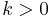$k > 0$ consists of a root node with$k$ children. One of these children is the root of a binomial tree with height 0, one is the root of a binomial tree with height 1, and so on up to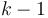$k-1$. When we merge two binomial trees of the same size, we simply make the smaller root the child of the larger root. Observe that this instantly gives a binomial tree of twice the size (whose height is then one greater than the heights of the two original trees). When we split a binomial tree of height$k > 0$, we simply detach the subtree of height$k-1$, giving two binomial trees of size$k-1$.

We could, in principle, convert a binomial tree of height$k$ into a power-of-two tree of height$k$ with the same keys, and vice versa. This is done recursively. If$k=0$, then we don't do anything, since a binomial tree of height 0 is a single node, and so is a power-of-two tree of height 0. If$k > 0$, then we split the binomial tree into two binomial trees of size$k-1$, recursively convert each one into its corresponding power-of-two tree, and then merge the results to get one power-of-two tree. The reverse procedure is analogous. This proves our claim that binomial trees and power-of-two trees are isomorphic.

In practice, power-of-two trees may be easier to work with than binomial trees because the former have a fixed maximum number of children per node (two) whereas the latter have to store linked lists of children in each node.

The names binomial tree and binomial heap derive from a cute property of binomial trees: the$k$th level of a binomial tree of height$n$ contains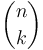$\binom{n}{k}$ nodes. This is easy to prove inductively; when merging two binomial trees, one of them is "shifted down" a level, so its nodes are added to the previous level of the other tree; this corresponds to the identity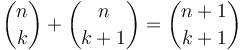$\binom{n}{k} + \binom{n}{k+1} = \binom{n+1}{k+1}$.